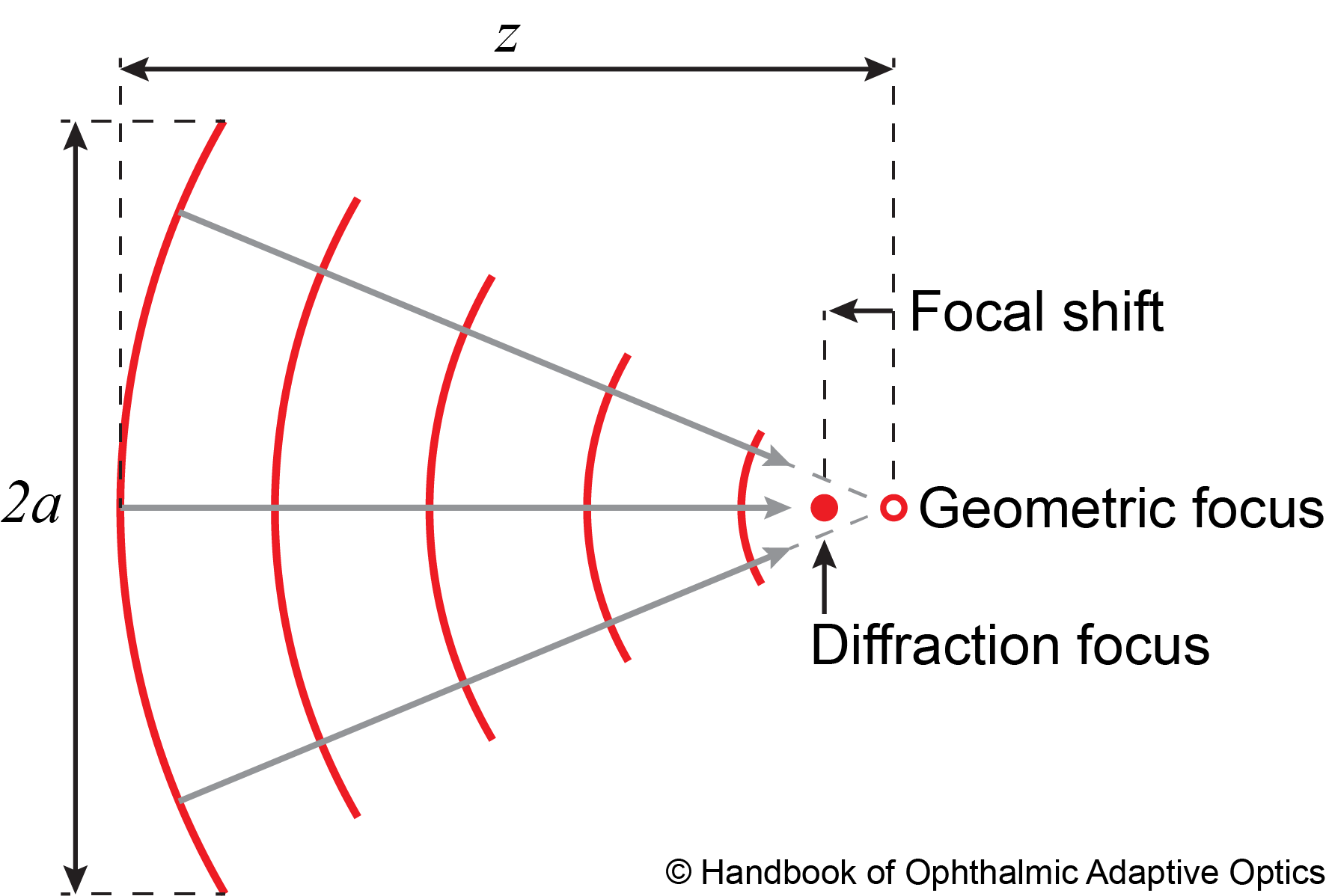### Focal shift

There are two points of interest near the focus of a converging spherical wavefront. The geometrical focus, where the rays orthogonal to the wavefront converge (see figure below), and the point of maximum intensity, which is shifted towards the incoming wavefront due to diffraction, and it is therefore referred to as the diffraction focus. The distance between these two foci, that is, the focal shift ($$\Delta z$$), is a function of the Fresnel number $$N = a^2 / \lambda z$$, where $$a$$ is the beam radius at a distance $$z$$ to the geometrical focus and $$\lambda$$ is the light wavelength.- Focal shift in a spherical wavefront (red lines= propagating from the left to right. The gray rays point towards the geometrical focus (hollow red spot), while the point of maximum intensity, the diffraction focus, is to the left (full red spot).

Yajun Li (Eq. 3.12) derived an approximate formula for the focal shift of a rotationally symmetric converging wavefront when $$N \geq 0.5$$ and $$F \geq 1$$,

\begin{equation} \Delta z_{L} \simeq - \frac{12 z \left(1 + \frac{1}{8F^2}\right)}{ \pi^2 N^2} \left\{ 1 - \exp \left[ -\frac{\pi^2 N^2}{12 \left( 1 + \frac{1}{8F^2} \right) \left[ 1 + N \left( 1- \frac{1}{16F^2}\right) \right]} \right]\right\}, \label{eq:Li_focal_shift_equation_name} \end{equation}

where the f-number of the beam, $$F = z / 2a$$. The negative sign indicates that the diffraction focus is always shifted towards the converging wavefront. Colin J. R. Sheppard and Peter Török (Eq. 17) arrived at a simpler formula for high numerical aperture systems with small focal shifts,

\begin{align*} \Delta z_{S-T} \simeq - \frac{z}{\cos^2(\alpha/2) + \left(\pi^2 N^2/12\right) \sec^2(\alpha/2)}, \end{align*}

where $$\alpha = \sin^{-1}(a/z)$$.

When a positive lens free of wavefront aberrations is normally illuminated with a plane wave, the Fresnel number, and thus the focal shift can be calculated n in terms of the lens clear aperture diameter $$D_L$$ and its back (geometrical) focal length $$f_L$$. In most imaging applications, however, the relative focal shift $$\Delta f_L / f_L$$ is negligible. For example, in a typical human eye $$f_{\textrm{eye}}\approx$$16.7 mm imaged with a conventional optical coherence tomgrapher $$D_{\textrm{beam}} \approx$$1.0 mm using 850 nm light, $$\Delta f_{\textrm{eye}} / f_{\textrm{beam}} \approx$$-0.4%. If, on the other hand, we consider a typical Shack-Hartmann wavefront sensor lenslet with 200 um diameter and 8 mm focal length, we have that the relative focal shift is approximately 29-36%, which is not negligible.